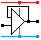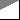Home

rOm
Quest
Glossary

Random
Page
Search
Site
Lush
Sim
Class
Subject
Images

Help
FAQ
Sign
Up
Log
In
 A Level     Audio     >Power and RMS<     Pre-Amplifier     Push-Pull     Tone Controls

# Audio Power and RMS

Site for Eduqas/WJEC - Go to the AQA site.

A Level    AudioQuestions 0 to 28   -->  View All

## DC Power

Power = Volts x Amps = VI        P = V2 / R        P = I2 R

This calculation is good for steady direct current (DC) levels and it also works for amplifiers producing a square wave output.

In a normal alternating current (AC) circuit the average voltage and current is less than the equivalent maximum DC level. The root mean square (RMS) values are used instead.

## AC, RMS - alternating current, root mean square

RMS AC power produces the same heating or lighting effect as DC power.

To get the average of an AC signal, first square the values to remove the minus sign from the negative parts of the AC waves. Then square root the value to get back to the original levels without the minus signs. Finally get the average or mean.

If you just took the average, it would always be zero due to the negative parts of the AC waves.

VRMS = VPeak / 1.414      (1.414 is the square root of 2)

VRMS = 0.707 VPeak        (0.707 = 1 / 1.414)

## RMS Output Power - Three formulas for RMS, Peak and Peak to Peak Voltage.

• The power supply is 20 Volts (Peak) or 40 Volts (Peak to Peak)
• An 8Ω speaker is being used.
• Decide whether to use 20V (ideal) or 18V (real life) in the calculation. If the exam question does not make it clear which one to use, just say whether you are doing the ideal or real life calculation. Below, the ideal calculation is shown.

VRMS     =    0.707 x VPeak    =    VPeak / √2

Power    =    VRMS2 / R          =    VPeak2 / ( 2 R )    =    VPeak to Peak2 / ( 8 R )

Power    = (20 x 0.707)2 / 8    =    202 / ( 2 x 8 )     =    402 / ( 8 x 8)    =    25 Watts

This is the theoretical maximum power output.

## Real Life Power Output

In real life, MOSFET push pull source followers are not perfect. The output will be lower than expected because ...

• The driver op-amp saturates a couple of volts below the power supply voltage.
• 2 or 3 volts are lost across the gate source junction in the MOSFETs.
• 0.7 to 4 Volts get lost in the biasing diodes depending on the type of diodes and transistors used.
• The MOSFETs have Drain to Source resistance. Energy is lost here.

Points 1 to 3 above can be fixed by running the op-amp driver and MOSFET biasing on a higher power supply voltage. As these are low power circuits, this is not too expensive to do.

Subject Name     Level     Topic Name     Question Heading     First Name Last Name Class ID     User ID

 CloseEscapeX Q: qNum of last_q     Q ID: Question ID         Score: num correct/num attempts         Date Done

Question Text

image url

Help Text
Debug

• You can attempt a question as many times as you like.
• If you are logged in, your first attempt, each day, is logged.
• To improve your scores, come back on future days, log in and re-do the questions that caused you problems.
• In the grade book, you can delete your answers for a topic before re-doing the questions. Avoid deleting unless you intend re-doing the questions very soon.